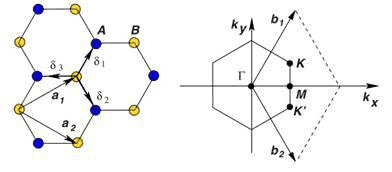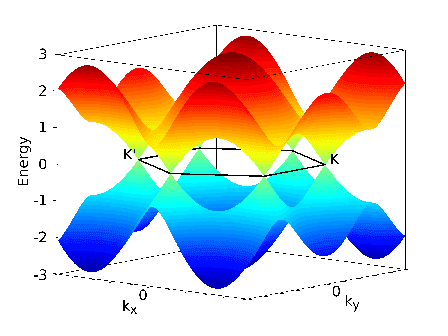# How to plot band structure?

I just learned how to get a tight-binding dispersion for graphene.
But i can not figure out how can I plot that. I want to plot that in the following path: Γ→K→M→Γ
I would be appreciated if could some one give me a hand on my problem.
thanks for alot.

ZapperZ
Staff Emeritus
I just learned how to get a tight-binding dispersion for graphene.
But i can not figure out how can I plot that. I want to plot that in the following path: Γ→K→M→Γ
I would be appreciated if could some one give me a hand on my problem.
thanks for alot.

What do you mean that you can't figure out how to plot the band?

If you are going along one particular axis, set one of the kx, let's say, set ky=0 and plug that in to your dispersion equation. If you are plotting along the zone diagonal, set kx=ky and off you go. Do the same for other high-symmetry directions.

Zz.

What do you mean that you can't figure out how to plot the band?

If you are going along one particular axis, set one of the kx, let's say, set ky=0 and plug that in to your dispersion equation. If you are plotting along the zone diagonal, set kx=ky and off you go. Do the same for other high-symmetry directions.

Zz.
Best wishes and Thank you again.
I have Hamiltonian matrix and overlap matrix. How to calculate tight binding dispersion for graphene in matlab?
I would be appreciated if could some one give me a hand on my problem.

DrDu
But ZapperZ told you already. Just take kx=ky=t and plot E(t).

Could you elaborate a little more? Is your question about the physics of tight binding calculations or about implementing tight binding calculations in MATLAB?

Could you elaborate a little more? Is your question about the physics of tight binding calculations or about implementing tight binding calculations in MATLAB?
implementing tight binding calculation in matlab.
matlab cose in the following path: Γ→K→M→Γ is:
Nk=20;
t=-2.550;
for ik=1:Nk+1
k_x=2*pi*(ik-1)/Nk*0;
k_y=2*pi*(ik-1)/Nk*0.667;
H=zeros(2);
H(1,2)=t*(exp(-1i*k_x/sqrt(3))+exp(1i/(2*sqrt(3))*((k_x+sqrt(3)*k_y)))+exp(1i/(2*sqrt(3))*(k_x-sqrt(3)*k_y)));
H(2,1)=t*(exp(1i*k_x/sqrt(3))+exp(-1i/(2*sqrt(3))*((k_x+sqrt(3)*k_y)))+exp(-1i/(2*sqrt(3))*(k_x-sqrt(3)*k_y)));
[V,D]=eig(H)
E_1(ik)=D(1,1)
E_2(ik)=D(2,2)
end
plot(E_1)
hold on
plot(E_2)
Nk=20;
t=-2.550;
for ik=21:Nk+10
k_x=2*pi*(ik-1)/Nk*0;
k_y=2*pi*(ik-1)/Nk*-0.667;
H=zeros(2);
H(1,2)=t*(exp(-1i*k_x/sqrt(3))+exp(1i/(2*sqrt(3))*((k_x+sqrt(3)*k_y)))+exp(1i/(2*sqrt(3))*(k_x-sqrt(3)*k_y)));
H(2,1)=t*(exp(1i*k_x/sqrt(3))+exp(-1i/(2*sqrt(3))*((k_x+sqrt(3)*k_y)))+exp(-1i/(2*sqrt(3))*(k_x-sqrt(3)*k_y)));
[V,D]=eig(H)
E_1(ik)=D(1,1)
E_2(ik)=D(2,2)
end
plot(E_1)
hold on
plot(E_2)
Nk=20;
t=-2.550;
for ik=31:Nk+20
k_x=2*pi*(ik-1)/Nk*0.557;
k_y=2*pi*(ik-1)/Nk*0;
H=zeros(2);
H(1,2)=t*(exp(-1i*k_x/sqrt(3))+exp(1i/(2*sqrt(3))*((k_x+sqrt(3)*k_y)))+exp(1i/(2*sqrt(3))*(k_x-sqrt(3)*k_y)));
H(2,1)=t*(exp(1i*k_x/sqrt(3))+exp(-1i/(2*sqrt(3))*((k_x+sqrt(3)*k_y)))+exp(-1i/(2*sqrt(3))*(k_x-sqrt(3)*k_y)));
[V,D]=eig(H)
E_1(ik)=D(1,1)
E_2(ik)=D(2,2)
end
plot(E_1)
hold on
plot(E_2)
but in the following path: K→Γ→M→K not true.
Nk is The number of points between two points K and Γ.

It looks like your not selecting the correct k points on your last for loop. If you're mainly interested in the band structure and not the wavefunctions you can cut through Γ,K, and M in one line, with M being in 2BZ, which is a direction similar to your first for loop. But if you want to look at different symmetry points, I usually define the coords of Γ,K,M, then define a vector that connects them like v1=K-Γ. I can't tell you if you've selected the correct symmetry points because that depends how you build your lattice

graphene lattice is:#### Attachments

ok. So if you set kx=0, and iterate ky you will plot through all the symmetry points. Also, K=(b1-b2)/3, K'=(2*b2+b1)/3, M=(b2+b1)/2. I would also suggest that you plot your path in k-space as a check.

ok. So if you set kx=0, and iterate ky you will plot through all the symmetry points. Also, K=(b1-b2)/3, K'=(2*b2+b1)/3, M=(b2+b1)/2. I would also suggest that you plot your path in k-space as a check.
Thank you very much.
I'm able to plot the 2D for graphene on matlab.
matlab code is:
close all
clear all
clc
t=-2.550;
acc=1.44e-10;
a=1.732*acc;

s=0:0.02:1
k_x=(2*pi/(sqrt(3)*a))*(1-s)
k_y=(2*pi/(3*a))*(1-s)
E1=t.*sqrt(1+4.*cos((sqrt(3).*a/2).*k_x).*cos((a/2).*k_y)+4.*cos((a/2).*k_y).^2)
E2=-E1;
plot(E1,'*')
hold on
plot(E2,'*')

s=0:0.02:1
k_x=(2*pi/(sqrt(3)*a))*s
k_y=0
E1=t.*sqrt(1+4.*cos((sqrt(3).*a/2).*k_x).*cos((a/2).*k_y)+4.*cos((a/2).*k_y).^2)
E2=-E1;
plot(E1,'*')
hold on
plot(E2,'*')

s=0:0.02:1
k_x=(2*pi/(sqrt(3)*a))
k_y=(2*pi/(3*a))*s
E1=t.*sqrt(1+4.*cos((sqrt(3).*a/2).*k_x).*cos((a/2).*k_y)+4.*cos((a/2).*k_y).^2)
E2=-E1;
plot(E1,'*')
hold on
plot(E2,'*')
but I can not draw diagrams tandem.

Just concatenate E = [E1 E2 E3]

Just concatenate E = [E1 E2 E3]
The problem was solved
thanks

Hi dear friends.
I'm able to plot graphene banstructure in 2D, by this code:
*****************************************************************************************
%Plot of Energy dispersion trial1.m
x=linspace(-2.5,2.5,50); y=linspace(-9,10,20);
[a,b]=meshgrid(x,y)
z= 3.033*sqrt(1+4*cos(b*0.1*pi).*cos(a*1.2283)+4*(cos(a*1.2283)).^2);
zz=- 3.033*sqrt(1+4*cos(b*0.1*pi).*cos(a*1.2283)+4*(cos(a*1.2283)).^2);
figure,plot(x,z)
title('Energy dispersion relation of graphene')
xlabel('K(1/A)'),ylabel(' Energy of graphene(ev)')
hold on
plot(x,zz)
***************************************************************************************
But now i'm trying to have graphene bandstructure in 3D like this:But I can't do this.
Can you give me the matlab code for 3D-Bandstructure?

@sina67 ... here's a modified code for 3d plot:
%Plot of Energy dispersion trial1.m
x=linspace(-2.5,2.5,50);
y=linspace(-9,10,20);
[a,b]=meshgrid(x,y)
z= 3.033*sqrt(1+4*cos(b*0.1*pi).*cos(a*1.2283)+4*(cos(a*1.2283)).^2);
zz=- 3.033*sqrt(1+4*cos(b*0.1*pi).*cos(a*1.2283)+4*(cos(a*1.2283)).^2);
figure
surf(a,b,z)
title('Energy dispersion relation of graphene')
xlabel('K(1/A)'),ylabel(' Energy of graphene(ev)')
hold on
surf(a,b,zz)

•Douasing
Can anyone write a matlab code for the 3D band structure from the Hamiltonian matrix? Not the one from the explicit expression for the eigenvalue, but just from the Hamiltonian to 3D plot. Thanks a lot!

Henryk
Gold Member
just a thought
Years ago I was working on graphite intercalation compounds. Did Fermi surface study. Then, made 2-D band structure calculations and found out that to get a good fit I had to include second nearest neighbour interactions within the tight binding model.

Barana, what is a and acc?